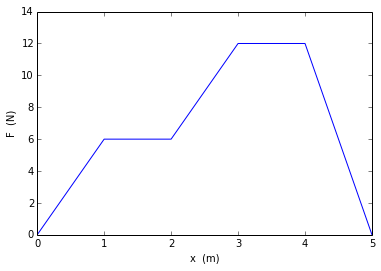# Chapter 2 Work, Energy and Power¶

## Example 2.1 Page no 26¶

In :
#given
F=(6,2)                                          #Constant force in vector form 6i+2j in N
s=(3,5)                                          #Displacement in vector form 3i+5j in N

#Calculations
import math
W=(F*s)+(F*s)
q=math.acos(W/(math.sqrt(F**2+F**2)*math.sqrt(s**2+s**2)))*180/3.14

#Output
print"Workdone by the force is ",W,"J"
print"Angle between Force and displacement is ",round(q,1),"degrees"

Workdone by the force is  28 J
Angle between Force and displacement is  40.6 degrees


## Example 2.2 Page no 26¶

In :
#given
m=10                                       #Mass of block in kg
q=40                                       #Angle made by the force with horizontal in degrees
s=5                                        #Horizontal displacement of the block in m
u=0.3                                      #Coefficient of kinematic friction

#Calculations
import math
F=(u*m*9.8)/(math.cos(q*3.14/180.0)+(u*math.sin(q*3.14/180.0)))
W=(F*math.cos(q*3.14/180.0))*s

#Output
print"Workdone by the pulling force is ",round(W,1),"J"

Workdone by the pulling force is  117.5 J


## Example 2.3 Page no 27¶

In :
#plot
import matplotlib.pyplot as plt
fig = plt.figure()
x=[0,1,2,3,4,5]
F=[0,6,6,12,12,0]
xlabel("x  (m)")
ylabel("F  (N)")
plt.xlim((0,5))
plt.ylim((0,14))
a=plot(x,F)
show(a)## Example 2.4 Page no 27¶

In :
#given
m=0.05                                                 #Mass of the body in kg
v=(3,5)                                                #Velocity in vector form 3i+4j in m/s

#Calculations
ke=(1/2.0)*m*(v**2+v**2)

#Output
print"Kinetic energy is ",ke,"J"

Kinetic energy is  0.85 J


## Example 2.5 Page no 27¶

In :
#given
k=50                                  #Spring force constant in N/m
x=-0.02                               #Length of compression in m

#Calculations
W=(1/2.0)*k*(x)**2

#Output
print"Work done by the spring when the block comes from the compressed position to the equilibrium position is ",W,"J"

Work done by the spring when the block comes from the compressed position to the equilibrium position is  0.01 J


## Example 2.6 Page no 27¶

In :
#given
x=0.03                                                 #Length stretched by the spring in m
m=0.25                                                 #Mass of the body in kg

#Calculations
k=(m*9.8)/x

#Output
print"Force constant of the spring is ",round(k,3),"N/m"

 Force constant of the spring is  81.667 N/m


## Example 2.7 Page no 27¶

In :
#given
m=5                                              #Mass of block in kg
F=20                                             #Constant force in N
x=6                                              #Distance moved by the block in m

#Calculations
import math
W=(F*x)
v=math.sqrt((2*W)/m)

#Output
print"Speed of the block when it moves through a distance of ",x,"m is",round(v,2),"m/s"

Speed of the block when it moves through a distance of  6 m is 6.93 m/s


## Example 2.8 Page no 28¶

In :
#given
m=50                                     #Mass of the object in kg
v=8                                      #Speed in m/s
t=4                                      #Time taken in s

#Calculations
a=(v-0)/t
s=(v**2/(2.0*a))
W=(m*a*s)
P=(W/t)

#Output
print"Workdone on the object is ",W,"J"
print"The average power delivered by the force in the first ",t,"s is ",P,"watt"

Workdone on the object is  1600.0 J
The average power delivered by the force in the first  4 s is  400.0 watt